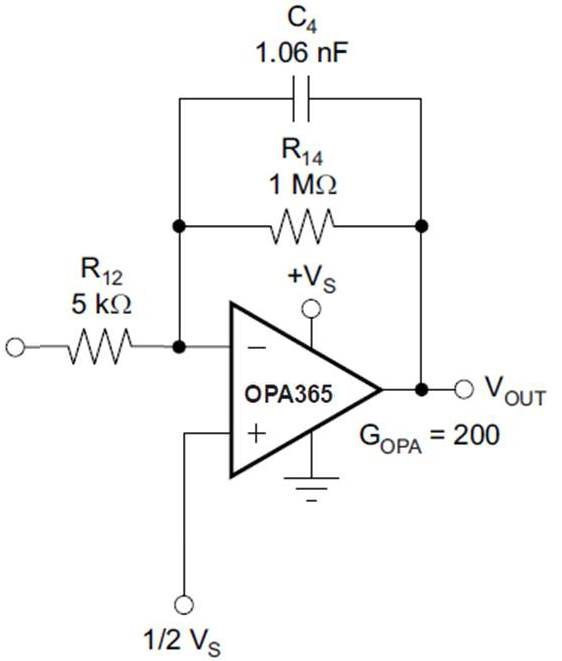# On Board with Bonnie: ADC noise from the inside out

Other Parts Discussed in Post: ADS1220

I must apologize. I am going to approach analog-to-digital converter (ADC) noise from an analog perspective. You may be surprised about this view because this discussion usually focuses on a digital perspective, but the analog outlook does work out quite elegantly.

Delta-sigma ADCs such as the ADS1220 (Figure 1) give you detailed information about generated data converter noise. The units of this noise description, en, are microvolts-rms (µVrms) and microvolts peak-to-peak (µVp-p).You can think of this noise as a referred-to-input phenomenon, similar to an amplifier (Figure 2). With the amplifier, the units of noise, en, are nanovolts per root hertz (nV/ÖHz). Over a specified bandwidth, the en units are microvolts-rms (µVrms). Another unit of measure for operational amplifier (op amp) noise is microvolts peak-to-peak (µVp-p).Figure 2: Op amp circuit with a closed-loop gain of –200 V/V

Let’s now look at the referred-to-output noise of a delta-sigma ADC and op amp. Both are closed-loop systems, where the ADC processes the signal with an internal digital filter and the amplifier has an external resistor network. In both cases, the devices send the output-noise signals to the output pin. In the case of the ADC, this would be the DOUT pin. In the case of the amplifier, this would be the VOUT pin (and you are looking for the total noise).

Typical specifications for the delta-sigma ADC’s output noise are effective-number-of-bits (ENOB) and noise-free-bits (NFb). Equations 1 and 2 are generic formulas for these two specifications:

ENOB = log[(2*VREF/GAIN)/( en_uVrms)] / log 2                   (1)

NFb = log[(2*VREF/GAIN)/(en_uVp-p)] / log 2                        (2)

where VREF is the data converter’s applied voltage reference and GAIN is the data converter’s gain, per an internal programmable gain amplifier (PGA).

These specifications are very important if you plan to compare ADC to ADC, but consider the power behind the noise specifications when determining the repeatability of your sensor system.

In Figure 1, the sensitivity of the load cell is 2 m/V and the maximum capacity (FSg) is 100 kg. For this system, the repeatability in grams (REPg) or minimum measurement value is equal to Equation 3:

REPg = en:p-p * FSg / (AVDD * Sensitivity)                 (3)

where AVDD is the voltage across the bridge as well as the ADS1220 positive analog supply.

For this ADC, at a data rate (DR) of 20 SPS, a PGA gain of 64 and in normal mode, FSg is equal to 100,000g and ADS1220 µVPP noise is 0.35 µV (per Table 1).

REPg = FSg (1 - en:p-p / (AVDD * sensitivity))

REPg = 0.35 µV * 100 kg / (5 V * 2 mV/V)

REPg = 0.7 gmTable 1: ADS1220 in µVRMS (µVPP), normal mode

The analog point of view is useful in the oddest places. With load cells and delta-sigma ADCs, you can stretch your imagination into the analog domain and use the specifications to your system’s advantage.